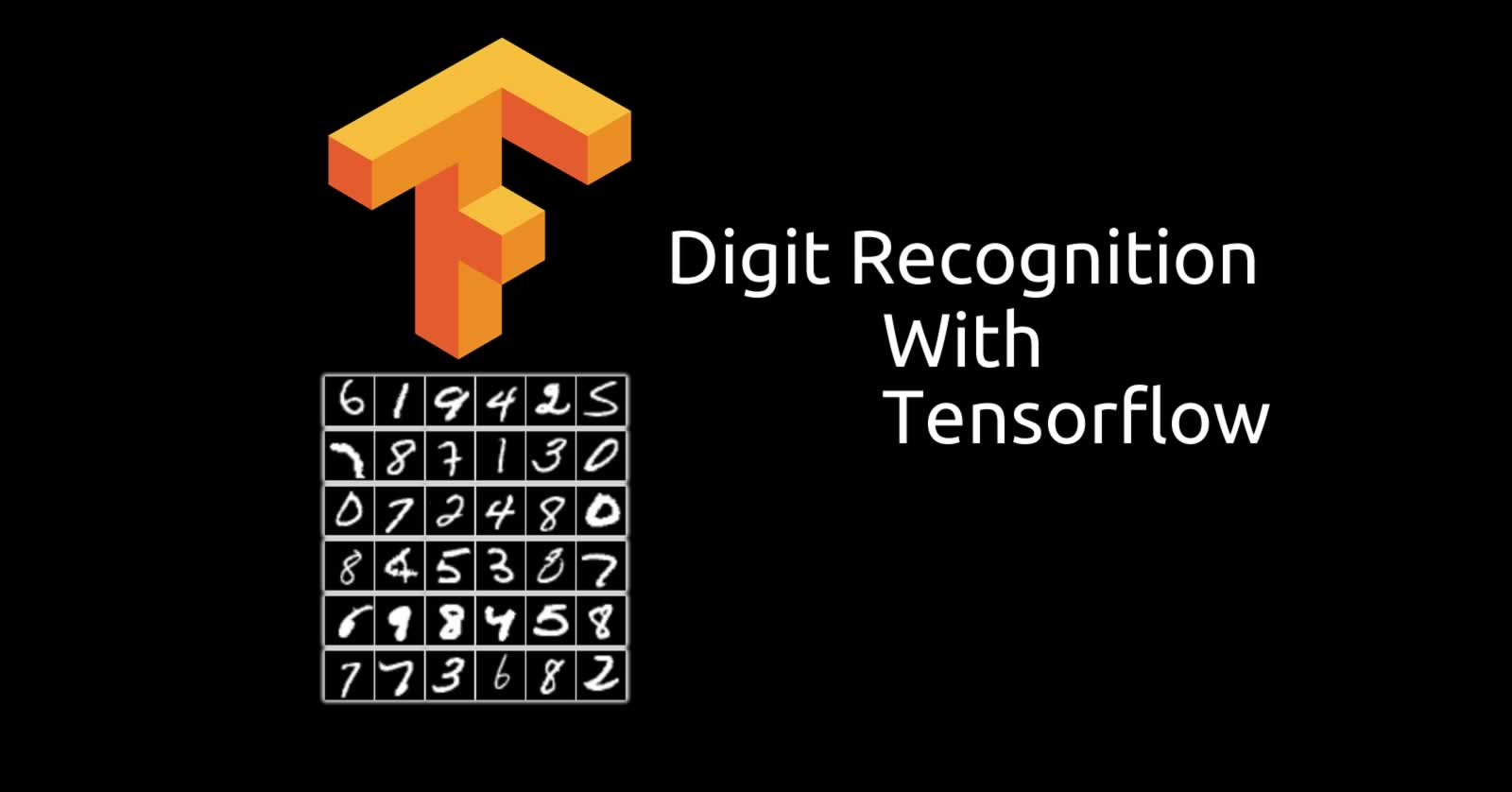# My first digit image recognition model

Written on Wed, 29 June 2016

I did it! I ran my first image recognition model that can read digits in images and accurately guess the the numbers. In all honesty, it was almost a copy and paste. The tutorial was the equivalent of a Hello World in programming. So really, nothing too impress to brag about.

Here's the codes anyway.

from tensorflow.examples.tutorials.mnist import input_data

import tensorflow as tf

x = tf.placeholder(tf.float32, [None, 784])

w = tf.Variable(tf.zeros([784, 10]))
b = tf.Variable(tf.zeros())

y = tf.nn.softmax(tf.matmul(x, w) + b)

y_ = tf.placeholder(tf.float32, [None, 10])
cross_entropy = tf.reduce_mean(-tf.reduce_sum(y_ * tf.log(y), reduction_indices=))

init = tf.initialize_all_variables()

sess = tf.Session()
sess.run(init)

for i in range(1000):
batch_xs, batch_ys = mnist.train.next_batch(100)
sess.run(train_step, feed_dict={x: batch_xs, y_: batch_ys})

correct_prediction = tf.equal(tf.argmax(y,1), tf.argmax(y_,1))
accuracy = tf.reduce_mean(tf.cast(correct_prediction, tf.float32))
print(sess.run(accuracy, feed_dict={x: mnist.test.images, y_: mnist.test.labels}))

I have no idea half of the things I typed. But hey, it worked.

Navigate
Related Content